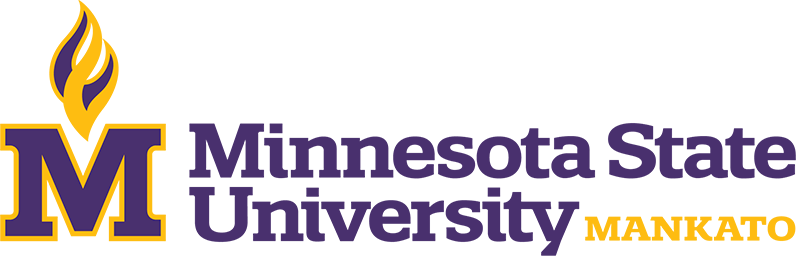Catalog Year

Degree

Credits

Locations

# Mathematics and Statistics (MS)

This program is designed to prepare students to pursue a Ph.D. program in either statistics or mathematics, or prepare students to work in business or industry.## Program Requirements

### Common Core

(0-16) credits

The topology of Euclidean spaces, norms, classical inequalities, local and global properties of continuous functions, preservation of compactness and connectedness, sequences in Euclidean space and sequences of functions.

Prerequisites: MATH 223 and MATH 290 with “C” (2.0) or better or consent.

An in-depth study of linear operators and their related spaces, dimension, rank, matrix representation of linear operators, special matrices, determinants, eigenvectors, and eigenvalues.

Prerequisites: MATH 345 with “C” (2.0) or better or consent.

This course provides an introduction to techniques and analysis involved with solving mathematical problems using technology. Topics included are errors in computation, solutions of linear and nonlinear equations, numerical differentiation and integration, and interpolation.

Prerequisites: MATH 122 and MATH 247 with “C” (2.0) or better or consent.

Simple and multiple regression, correlation, analysis of variance and covariance.

Prerequisites: MATH 354 or STAT 354 or (MATH 455 or MATH 555) or (STAT 455 or STAT 555) with “C” (2.0) or better or consent.

A mathematical approach to statistics with derivation of theoretical results and of basic techniques used in applications. Includes probability, continuous probability distributions, multivariate distributions, functions of random variables, central limit theorem, and statistical inference. Same as MATH 555.

Prerequisites: MATH 223 with “C” (2.0) or better or consent.

A mathematical approach to statistics with derivation of theoretical results and of basic techniques used in applications, including sufficient statistics, additional statistical inference, theory of statistical tests, inferences about normal models, and nonparametric methods. Same as MATH 556.

Prerequisites: (MATH 455 or MATH 555) or (STAT 455 or STAT 555) with “C” (2.0) or better or consent.

### Unrestricted Electives

A minimum of 6 credits of 600 level Mathematics and a minimum of 6 credits of 600 level statistics. Advisor approval is required.

### Other Graduation Requirements

Thesis or Alternate Plan Paper: (1-4 credits) Math 694 Alternate Plan Paper (1-2) Math 699 Thesis (1-4) Comprehensive Exam: Four courses, two chosen from MATH 517, MATH 547 and MATH 570, and two chosen from STAT 550, STAT 555, STAT 556. Advisor approval is required.